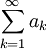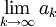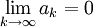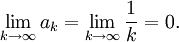# The Divergence Test

Previous: A Useful Theorem

Next: A Divergence Test Flowchart

Theorem 1 immediately yields the divergence test.

Theorem: The Divergence Test

Given the infinite series,if the following limitdoes not exist or is not equal to zero, then the infinite seriesmust be divergent.

No proof of this result is necessary: the Divergence Test is equivalent to Theorem 1.

If it seems confusing as to why this would be the case, the reader may want to review the appendix on the divergence test and the contrapositive.

### Beware: The Converse is Not Necessarily True

Observe that the converse of Theorem 1 is not true in general. Ifwe cannot conclude that the infinite series is convergent! For example, the harmonic series has the property thatBut the harmonic series is not a convergent series, as was shown in a an earlier section in the lesson on the harmonic and telescoping series.

Therefore, if the limit is equal to zero, the Divergence Test yields no conclusion: the infinite series may or may not converge. In this case, other convergence tests can be used to try to determine whether or not the series converges, if required.

Previous: A Useful Theorem

Next: A Divergence Test Flowchart